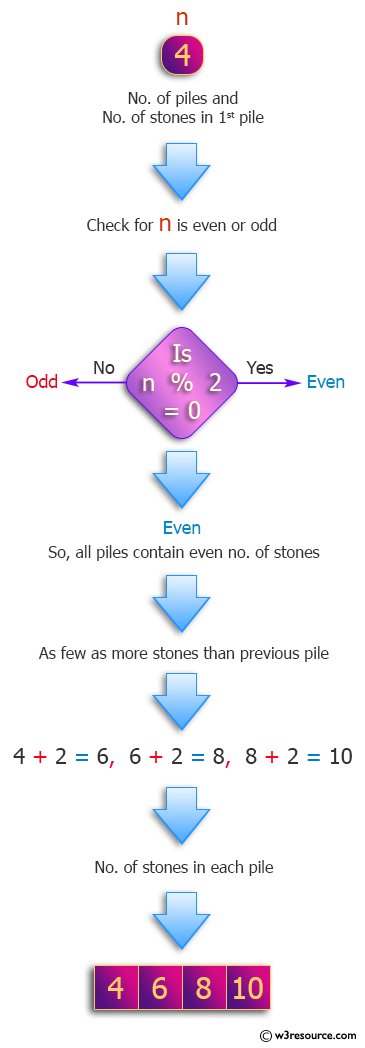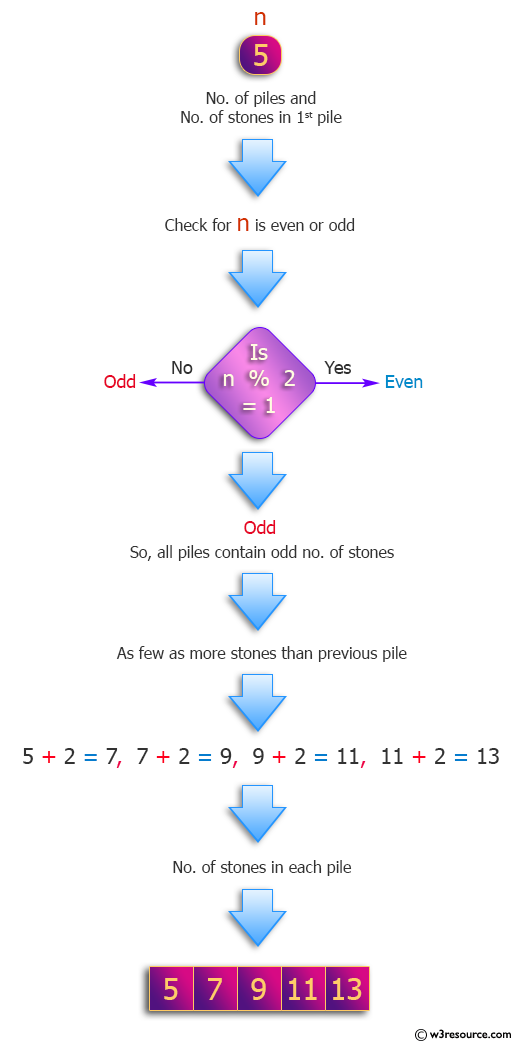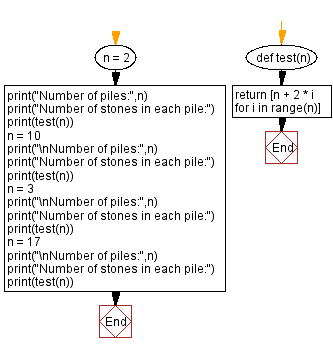﻿ Python: Find the number of stones in each pile - w3resource# Python: Find the number of stones in each pile

## Python Programming Puzzles: Exercise-4 with Solution

We are making n stone piles! The first pile has n stones. If n is even, then all piles have an even number of stones. If n is odd, all piles have an odd number of stones. Each pile must more stones than the previous pile but as few as possible. Write a Python program to find the number of stones in each pile.

```Input:  2
Output:
[2, 4]

Input:  10
Output:
[10, 12, 14, 16, 18, 20, 22, 24, 26, 28]

Input: 3
Output:
[3, 5, 7]

Input: 17
Output:
[17, 19, 21, 23, 25, 27, 29, 31, 33, 35, 37, 39, 41, 43, 45, 47, 49]
```

Pictorial Presentation:Sample Solution:

Python Code:

``````#License: https://bit.ly/3oLErEI

def test(n):
return [n + 2 * i for i in range(n)]
n = 2
print("Number of piles:",n)
print("Number of stones in each pile:")
print(test(n))
n = 10
print("\nNumber of piles:",n)
print("Number of stones in each pile:")
print(test(n))
n = 3
print("\nNumber of piles:",n)
print("Number of stones in each pile:")
print(test(n))
n = 17
print("\nNumber of piles:",n)
print("Number of stones in each pile:")
print(test(n))
``````

Sample Output:

```Number of piles: 2
Number of stones in each pile:
[2, 4]

Number of piles: 10
Number of stones in each pile:
[10, 12, 14, 16, 18, 20, 22, 24, 26, 28]

Number of piles: 3
Number of stones in each pile:
[3, 5, 7]

Number of piles: 17
Number of stones in each pile:
[17, 19, 21, 23, 25, 27, 29, 31, 33, 35, 37, 39
```

Flowchart:## Visualize Python code execution:

The following tool visualize what the computer is doing step-by-step as it executes the said program:

Python Code Editor :

Have another way to solve this solution? Contribute your code (and comments) through Disqus.

What is the difficulty level of this exercise?

Test your Programming skills with w3resource's quiz.

﻿

## Python: Tips of the Day

Clamps num within the inclusive range specified by the boundary values x and y:

Example:

```def tips_clamp_num(num,x,y):
return max(min(num, max(x, y)), min(x, y))
print(tips_clamp_num(2, 4, 6))
print(tips_clamp_num(1, -1, -6))
```

Output:

```4
-1
```